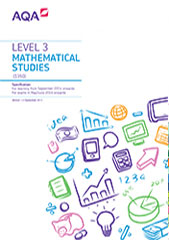# 3.7 Correlation and regression

## S7 Correlation

Content

S7.1

recognising when pairs of data are uncorrelated, correlated, strongly correlated, positively correlated and negatively correlated

S7.2

appreciating that correlation does not necessarily imply causation

S7.3

understanding the idea of an outlier

identifying and understanding outliers and make decisions whether or not to include them when drawing a line of best fit

## S8 The product moment correlation coefficient (pmcc)

Content

S8.1

understanding that the strength of correlation is given by the pmcc

S8.2

understanding that pmcc always has a value in the range from – 1 to + 1

S8.3

appreciating the significance of a positive, zero or negative value of pmcc in terms of correlation of data

## S9 Regression lines

Content

S9.1

the plotting of data pairs on scatter diagrams and the drawing, by eye, of a line of best fit through the mean point

the idea of residuals will not be required

S9.2

understanding the concept of a regression line

S9.3

plotting a regression line from its equation

S9.4

using interpolation with regression lines to make predictions

S9.5

understanding the potential problems of extrapolation

## S10 Calculations

Content